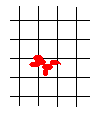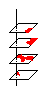Shapes in a lattice

ProblemImagine a rectangular grid (lattice in the math parlance) with the distance between the nodes equal to 1. Also, let there be given a shape with the area less than 1 but otherwise arbitrary. Show that it's possible to place this shape onto the plane in such a manner that no grid nodes will fall inside the shape.

SolutionImagine a rectangular grid (lattice in the math parlance) with the distance between the nodes equal to 1. Also, let there be given a shape with the area less than 1 but otherwise arbitrary. Show that it's possible to place this shape onto the plane in such a manner that no grid nodes will fall inside the shape.

Solution

There are two ways to look at the problem. As the problem was actually formulated, one starts with a lattice and fits a given shape trying to avoid the nodes. The second way is to place the shape onto the plane and then draw a grid around it so that no nodes will fall inside the shape. We may also combine the two: place the shape onto the given lattice, and then, if necessary, shift the lattice a little to avoid intersections.I'll pursue the latter course. After placing the shape on top of the grid, cut the plane into squares along the grid lines. (We already used such a trick while Shredding the torus.) Stack the squares (without rotating them) on top of each other. Let the plane be transparent and the shape opaque. Take now a look from the top of the stack down. What you'll see is an outline of a single square with a blot formed by the intersection of several pieces of the given shape that fell into different squares. The area of this intersection is clearly less than 1. Therefore, the blot can't cover the whole square. There must be a point that does not belong to the blot. Pierce the whole stack with a vertical line through this point. Now disassemble the stack and place the squares back into their original positions on the plane. Each of the squares will contain a point (the trace of the vertical line) none of which belongs to the shape. The collection of these points forms a new grid of side 1. This may be looked at as the new position of the old grid. Slide it there.Logic and math logic, in particular, might be flawless, and yet lead to a wrong result. This may happen if one starts with a faulty premise. I do not say that what we obtained is wrong. However, the whole formulation has relied on an intuitive notion of a planar shape and its area. I just want to draw your attention to the fact that our intuition may not be sufficient basis for mathematical derivations.

Let's ask ourselves a few questions?

Is a shape a set? Yes, probably. In so far as everything may be declared a set.

A set of what? It's a set of points, a point set.

Is every point set a shape?

It's a hard question and a source of our quandary. Let the lattice be associated with the integer grid lines of a coordinate system with the origin at one of the nodes. Consider a set of all points (x, y) with rational coordinates. Does this set have a shape? There is only a countable number of such pairs. Sometimes (in the theory of Generalized Functions) it's convenient to ascribe a positive measure to a single point. Measure is a rigorous math notion that formalizes intuitive numerical properties of geometric objects, like length, area, and volume. (I've mentioned the Hausdorff measures when talking about Fractal Dimension. However, talking of areas, having a point with a positive area is completely counterintuitive. So that we should assume that area as a measure, vanishes at every point.

Now, a measure may be expected to have some additive properties. We would expect, for example, that the area of two nonintersecting pieces is the sum of the component areas. In the Measure Theory, we are looking into infinite (but only countably infinite) sums (also known as series.) Thus the area of a countable set of points is also 0. Note that we can't apply the same reasoning to an uncountable set. If we did, then all and every set would have a zero area. Not very interesting.

If you go ahead and apply the stacking procedure to the above set of points with rational coordinates then the blot will actually appear to cover the whole of the square. We, of course, know that it's only an appearance. The set is countable while the square is not. Therefore, we are assured of existence of a point through which we would be able to pierce the stack.

What if we take the set of points (x, y) inside a single square with at least one coordinate irrational. By the additive property, we know (or, at least, expect) the area of such a set to be 1. There are still holes.

As the third example, let's consider a set that consists of two parts. The set of points with rational coordinates in one square plus the set of points with at least one irrational coordinate in another square. The stack in this case will have no holes.

May we be sure that it's impossible to remove a subset from the second part and augment the first part a little bit so that at the end we would have a weird set of area less than 1 with the union of two (shifted) halves covering the entire square? The answer to this question is a firm "No" - no, it's impossible to do that with just two parts. But who will vouch if we allow an infinite number of small parts?

Well, you should not worry about this either. If the set of measure less than 1 is spread over a number (perhaps, infinite) of squares then piling them on top of each other will create a set of measure less than 1 inside a single square so that it won't be able to cover a whole square whose measure is 1. However, the musings above might give you an idea why it is at least desirable to develop rigorous definitions for such simple and mundane notions as shape and area.